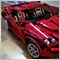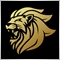# Array out of range error for not existing bars29

Hi,

I wrote a code to calculate the sum of past bar bodies. But in some charts, there is no bar in specific time, like silver/usd. I attached the chart.

This is the function I get the error in:

```double AvBarSize (int BarAmount)
{
double SumBar=0;
double ABSize;
int i;
for (i=3;i<BarAmount;i++)
{
SumBar = SumBar + MathAbs (Close[i]-Open[i]);
}
ABSize = SumBar / (BarAmount-3);
return (ABSize);
}```

When I want to execute this function on March 30, the bars doesn't exist as you seen in the chart and I get that error. What do you suggest not to get this error?

Files:393

Afshid:

Hi,

I wrote a code to calculate the sum of past bar bodies. But in some charts, there is no bar in specific time, like silver/usd. I attached the chart.

This the function I get the error in:

When I want to execute this function on March 30, the bars doesn't exist as you seen in the chart and I get that error. What do you suggest not to get this error?

You probably should compare BarAmount with Bars to make sure it is smaller...29

Seng Joo Thio:

You probably should compare BarAmount with Bars to make sure it is smaller...

Yes, Thank you. I think it works. But another question:

This helps if we want to know if the Bars count is equal to what we expect and the equation executes. But if we expect 200 Bars and we have 150 Bars, and we want to calculate on how many Bars we have (150 Bars) , What should we do? I want to skip non-existence Bars.393

Afshid:

Yes, Thank you. I think it works. But another question:

This helps if we want to know if the Bars count is equal to what we expect and the equation executes. But if we expect 200 Bars and we have 150 Bars, and we want to calculate on how many Bars we have (150 Bars) , What should we do? I want to skip non-existence Bars.

You can use the MathMin function, like this:

```   for (i=3;i<MathMin(Bars,BarAmount);i++)

ABSize = SumBar / (MathMin(Bars,BarAmount)-3);
```29

Seng Joo Thio:

You can use the MathMin function, like this:

Yes, It can count the existing Bars, but the error appears in here:

`SumBar = SumBar + MathAbs (Close[i]-Open[i]);`

how can I skip and do not try to calculate the missing Bar?393

Afshid:

Yes, It can count the existing Bars, but the error appears in here:

how can I skip and do not try to calculate the missing Bar?

When i ranges from >=3 to <MathMin(Bars,BarAmount), that statement should not cause any error.

If it still does, the way to debug will be to add some Print statements to see the values of BarAmount, Bars and i right before the error appears.

(btw, this is mql4 right?)29

Seng Joo Thio:

When i ranges from >=3 to <MathMin(Bars,BarAmount), that statement should not cause any error.

If it still does, the way to debug will be to add some Print statements to see the values of BarAmount, Bars and i right before the error appears.

(btw, this is mql4 right?)

Yes, it is mql4. I have debugged it before, and I realized that in some Times, there is no Bar. So when I refer as Open[i], it makes an run-time error, because there is no Bar there to extract its data. This is the problem.

I want to use something like this:

`if (Bar(i) exists) SumBar = SumBar + MathAbs (Close[i]-Open[i]);`393

Afshid:

Yes, it is mql4. I have debugged it before, and I realized that in some Times, there is no Bar. So the when I refer as Open[i], it makes an run-time error, because there is no Bar there to extract its data. This is the problem.

I want to use something like this:

What if, instead of Open[i]/Close[i], use iOpen()/iClose() instead? These functions will return value 0 if the bar does not exist - this way you can do a filtering, if necessary, of the return values before incorporating them into your calculation.29

Seng Joo Thio:

What if, instead of Open[i]/Close[i], use iOpen()/iClose() instead? These functions will return value 0 if the bar does not exist - this way you can do a filtering, if necessary, of the return values before incorporating them into your calculation.

No, The problem is the [i] itself. You can not use Open[i], Close[i] or anything about [i]. Cause it doesn't exist.393

Afshid:
No, The problem is the [i] itself. You can not use Open[i], Close[i] or anything about [i]. Cause it doesn't exist.

You don't understand.

'i' will cause error when you use it access an array directly (when i is out of range). But iOpen() and iClose() are function calls, and within that function calls, all necessary safeguards were incorporated to prevent error condition so that the return is 0 (worst case) rather than error.Moderator
10735

You have to check on forehand if the bar exists and when it does you can continue and read it's value.

But when it does not exist don't try to read it because it will go out of range and unload the indicator.

To add comments, please log in or register